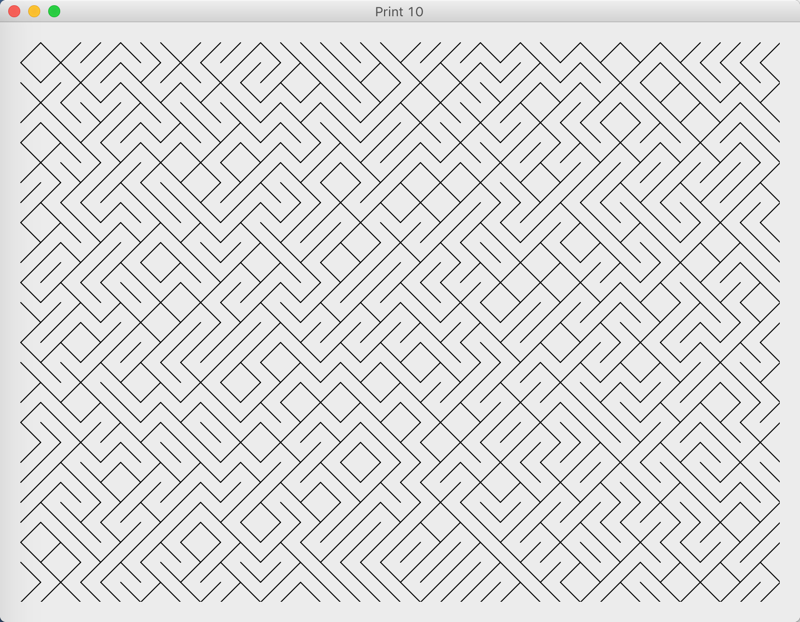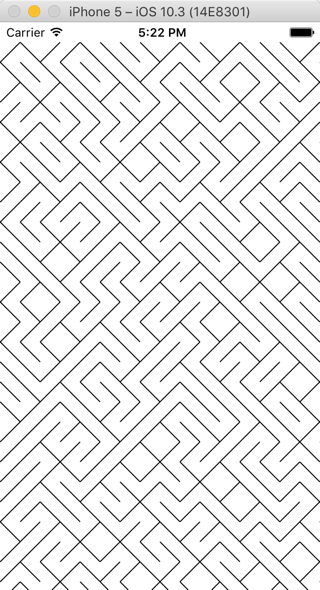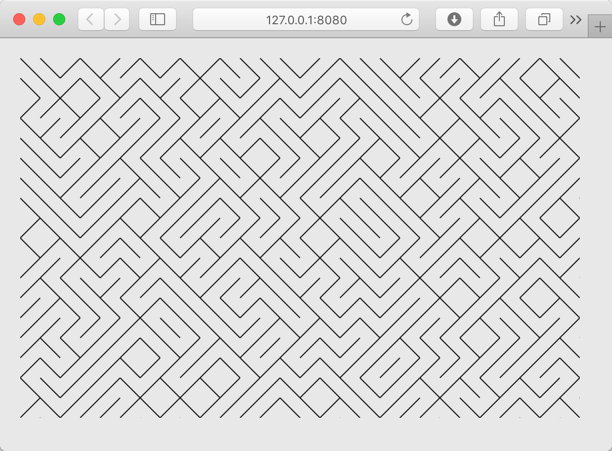The other day I saw this article on Dev.To: A Universe in One Line of Code with 10 PRINT

It talks about how you could make a maze-like structure on a Commodore 64 with just this one line of code:

`10 PRINT CHR\$(205.5+RND(1)); : GOTO 10`

The author then goes on to show you how you might do something similar using Python and pygame.

I love all things retro and this seemed like fun, so I thought I would whip up the same thing in Xojo.

For reference, the primary Python code looks like this (although there is more code to set up the drawing area):

```square = 20
for x in range(0, screenWidth, square):
for y in range(0, screenHeight, square):
if random.random() > 0.5:
pygame.draw.line(screen, white, (x, y), (x + square, y + square))
else:
pygame.draw.line(screen, white, (x, y + square), (x + square, y))```

To do this in Xojo, create a Desktop, web or iOS project, add a Canvas to the layout and put this code in its Paint event:

```Const kSquare = 20
For x As Integer = 0 To g.Width Step kSquare
For y As Integer = 0 To g.Height Step kSquare
If Rnd > 0.5 Then
g.DrawLine(x, y, x + kSquare, y + kSquare)
Else
g.DrawLine(x, y + kSquare, x + kSquare, y)
End If
Next
Next```

(Yes, for this example Xojo uses the exact same code for desktop, web and iOS.)

You should certainly be able to see the similarities between Xojo and Python. Here’s the output of Xojo desktop, web and iOS apps:Be sure to check out Dev.To and follow me there as well.